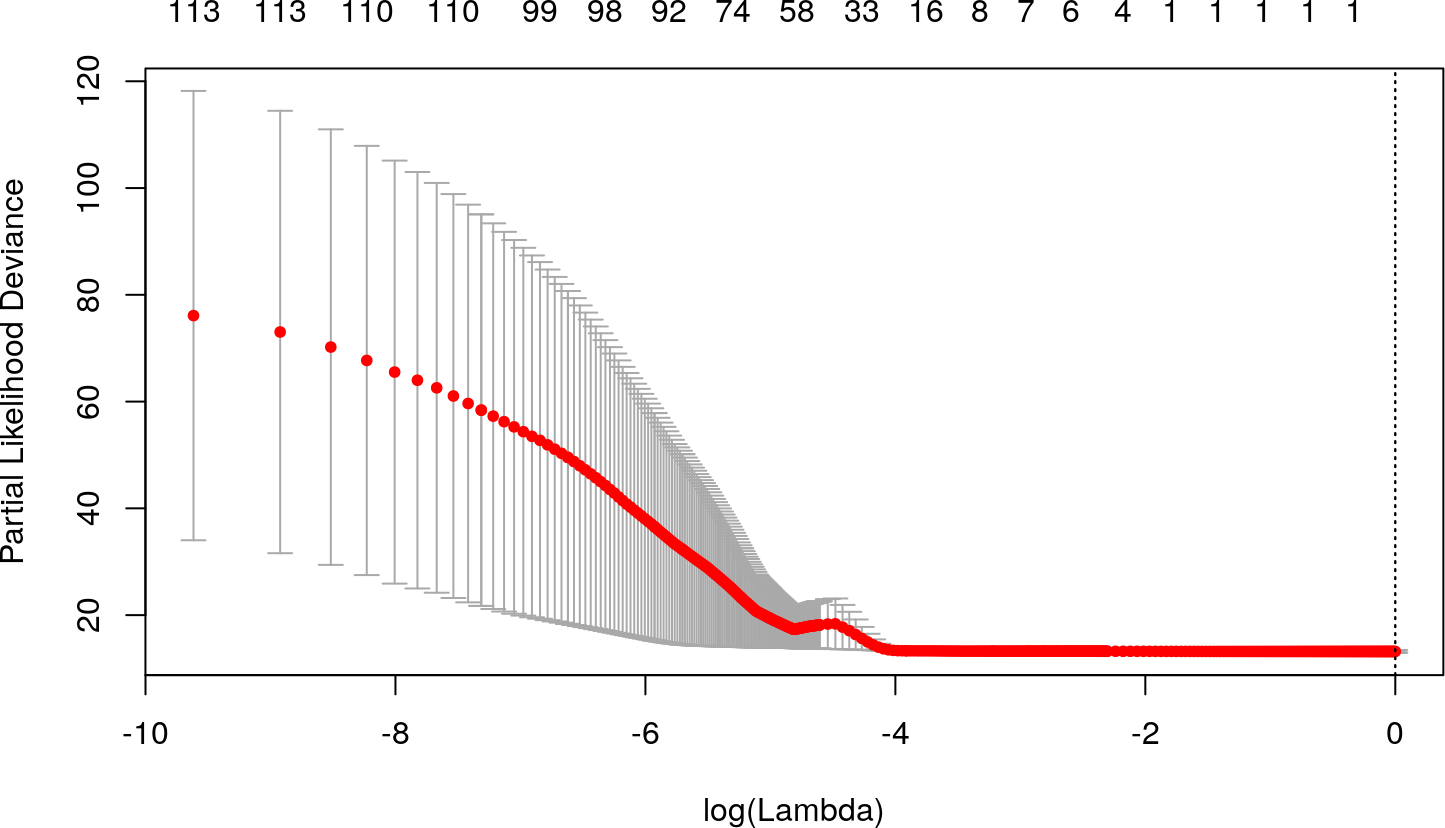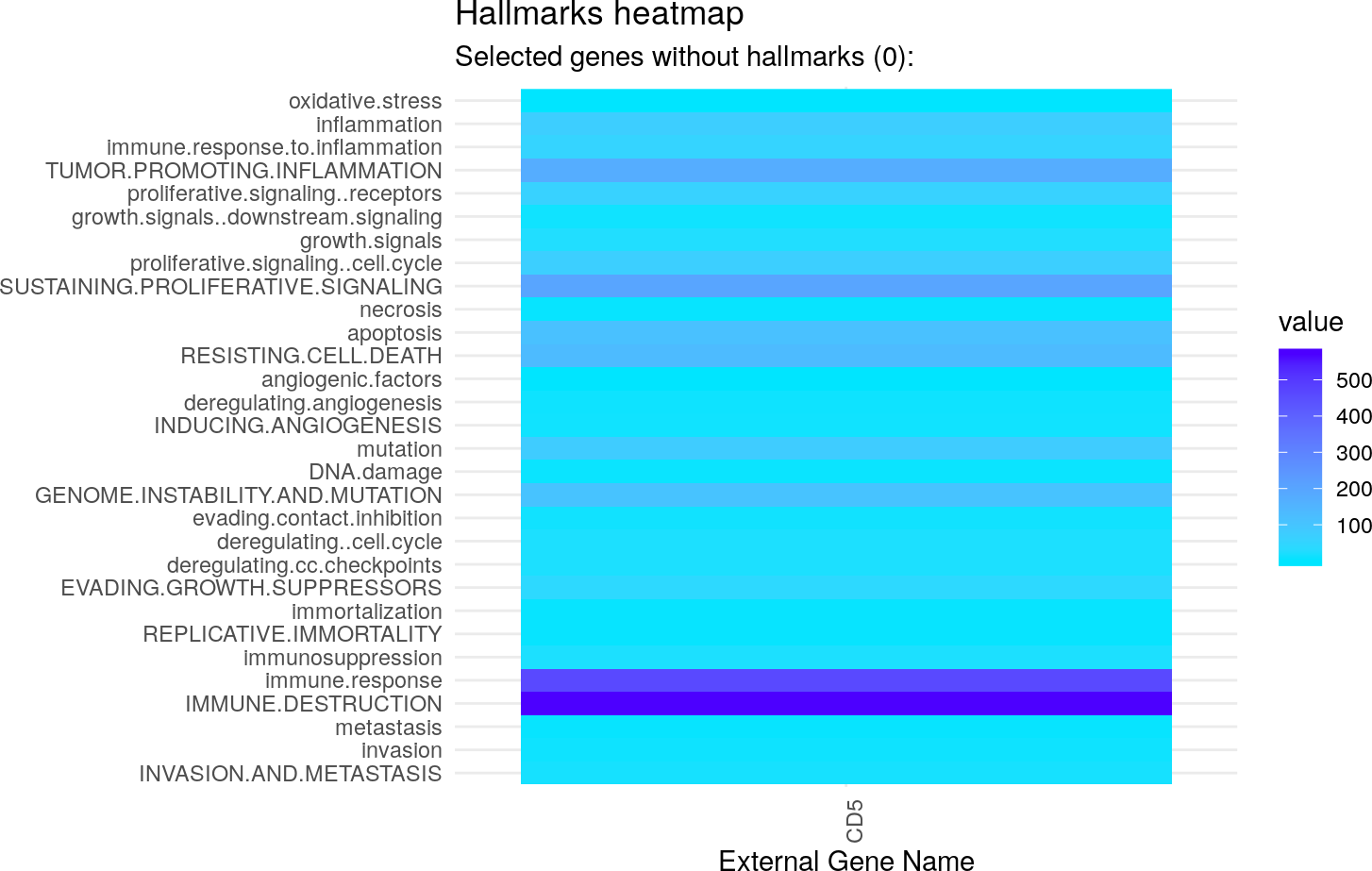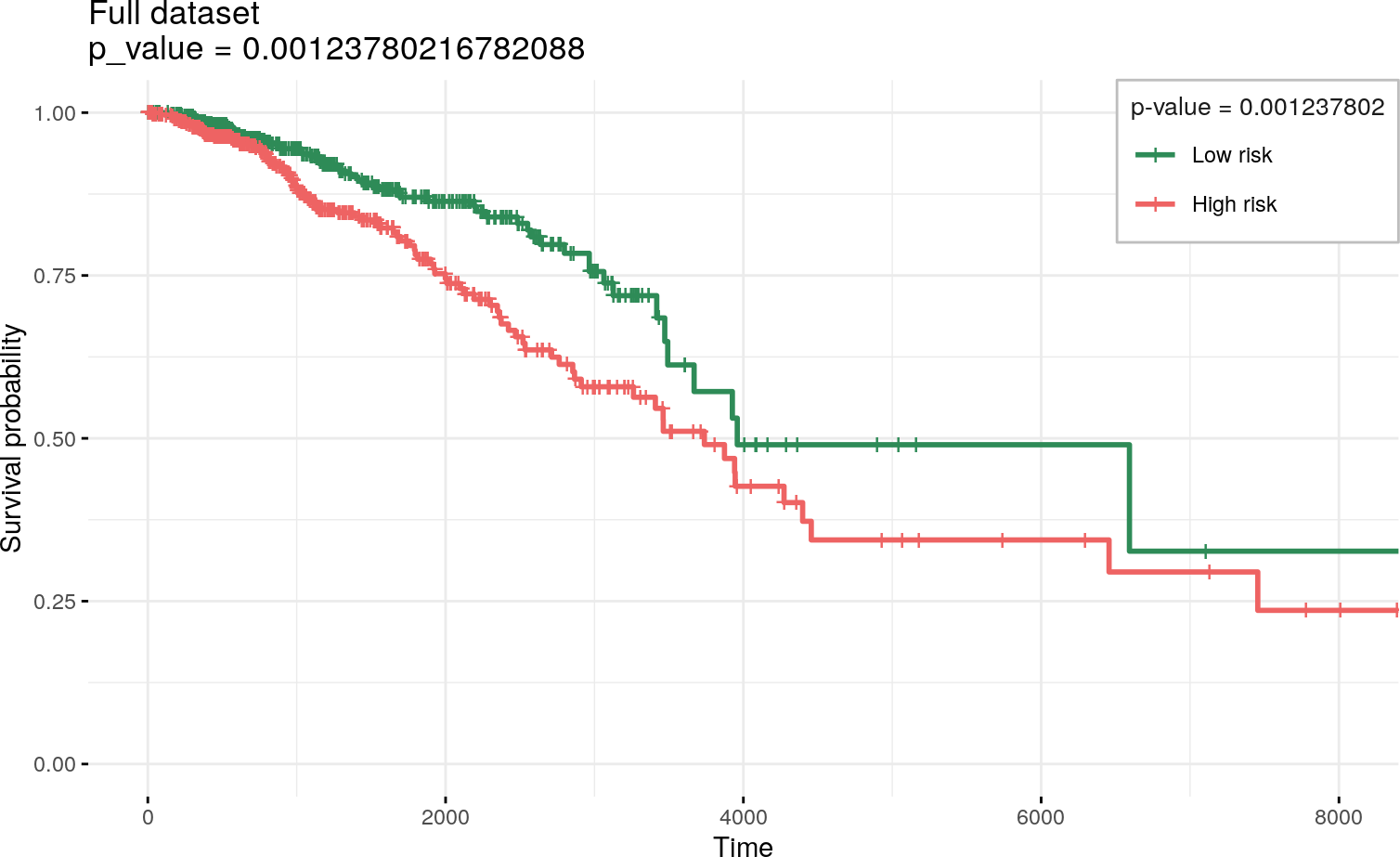# Contents

## 0.1 Instalation

if (!require("BiocManager"))
install.packages("BiocManager")
BiocManager::install("glmSparseNet")

# 1 Required Packages

library(dplyr)
library(ggplot2)
library(survival)
library(loose.rock)
library(futile.logger)
library(TCGAutils)
#
library(glmSparseNet)
#
# Some general options for futile.logger the debugging package
.Last.value <- flog.layout(layout.format('[~l] ~m'))
.Last.value <- loose.rock::show.message(FALSE)
# Setting ggplot2 default theme as minimal
theme_set(ggplot2::theme_minimal())

The data is loaded from an online curated dataset downloaded from TCGA using curatedTCGAData bioconductor package and processed.

To accelerate the process we use a very reduced dataset down to 107 variables only (genes), which is stored as a data object in this package. However, the procedure to obtain the data manually is described in the following chunk.

brca <- curatedTCGAData(diseaseCode = "BRCA", assays = "RNASeq2GeneNorm", FALSE)
# keep only solid tumour (code: 01)
brca.primary.solid.tumor <- TCGAutils::splitAssays(brca, '01')
xdata.raw <- t(assay(brca.primary.solid.tumor[]))

# Get survival information
ydata.raw <- colData(brca.primary.solid.tumor) %>% as.data.frame %>%
# Keep only data relative to survival or samples
select(patientID, vital_status,
Days.to.date.of.Death, Days.to.Date.of.Last.Contact,
days_to_death,         days_to_last_followup,
Vital.Status) %>%
# Convert days to integer
mutate(Days.to.date.of.Death = as.integer(Days.to.date.of.Death)) %>%
mutate(Days.to.Last.Contact  = as.integer(Days.to.Date.of.Last.Contact)) %>%
# Find max time between all days (ignoring missings)
rowwise %>%
mutate(time = max(days_to_last_followup,        Days.to.date.of.Death,
Days.to.Last.Contact, days_to_death, na.rm = TRUE)) %>%
# Keep only survival variables and codes
select(patientID, status = vital_status, time) %>%
# Discard individuals with survival time less or equal to 0
filter(!is.na(time) & time > 0) %>% as.data.frame

# Set index as the patientID
rownames(ydata.raw) <- ydata.raw$patientID # Get matches between survival and assay data xdata.raw <- xdata.raw[TCGAbarcode(rownames(xdata.raw)) %in% rownames(ydata.raw),] xdata.raw <- xdata.raw %>% { (apply(., 2, sd) != 0) } %>% { xdata.raw[, .] } %>% scale # Order ydata the same as assay ydata.raw <- ydata.raw[TCGAbarcode(rownames(xdata.raw)), ] # Using only a subset of genes previously selected to keep this short example. set.seed(params$seed)
small.subset <- c('CD5', 'CSF2RB', 'IRGC', 'NEUROG2', 'NLRC4', 'PDE11A',
'PTEN', 'TP53', 'BRAF',
'PIK3CB', 'QARS', 'RFC3', 'RPGRIP1L', 'SDC1', 'TMEM31',
'YME1L1', 'ZBTB11', sample(colnames(xdata.raw), 100)) %>%
unique

xdata <- xdata.raw[, small.subset[small.subset %in% colnames(xdata.raw)]]
ydata <- ydata.raw %>% select(time, status)

# 3 Fit models

Fit model model penalizing by the hubs using the cross-validation function by cv.glmHub.

set.seed(params$seed) fitted <- cv.glmHub(xdata, Surv(ydata$time, ydata$status), family = 'cox', lambda = buildLambda(1), network = 'correlation', network.options = networkOptions(cutoff = .6, min.degree = .2)) ## Warning in regularize.values(x, y, ties, missing(ties)): collapsing to unique ## 'x' values ## Warning in regularize.values(x, y, ties, missing(ties)): collapsing to unique ## 'x' values ## Warning in regularize.values(x, y, ties, missing(ties)): collapsing to unique ## 'x' values ## Warning in regularize.values(x, y, ties, missing(ties)): collapsing to unique ## 'x' values ## Warning in regularize.values(x, y, ties, missing(ties)): collapsing to unique ## 'x' values ## Warning in regularize.values(x, y, ties, missing(ties)): collapsing to unique ## 'x' values ## Warning in regularize.values(x, y, ties, missing(ties)): collapsing to unique ## 'x' values ## Warning in regularize.values(x, y, ties, missing(ties)): collapsing to unique ## 'x' values ## Warning in regularize.values(x, y, ties, missing(ties)): collapsing to unique ## 'x' values ## Warning in regularize.values(x, y, ties, missing(ties)): collapsing to unique ## 'x' values # 4 Results of Cross Validation Shows the results of 100 different parameters used to find the optimal value in 10-fold cross-validation. The two vertical dotted lines represent the best model and a model with less variables selected (genes), but within a standard error distance from the best. plot(fitted)## 4.1 Coefficients of selected model from Cross-Validation Taking the best model described by lambda.min coefs.v <- coef(fitted, s = 'lambda.min')[,1] %>% { .[. != 0]} ## Warning in regularize.values(x, y, ties, missing(ties)): collapsing to unique ## 'x' values coefs.v %>% { data.frame(gene.name = names(.), coefficient = ., stringsAsFactors = FALSE) } %>% arrange(gene.name) %>% knitr::kable() gene.name coefficient CD5 -0.1663218 ## 4.2 Hallmarks of Cancer names(coefs.v) %>% { hallmarks(.)$heatmap }## 4.3 Survival curves and Log rank test

separate2GroupsCox(as.vector(coefs.v),
xdata[, names(coefs.v)],
ydata,
plot.title = 'Full dataset', legend.outside = FALSE)
## $pvalue ##  0.001237802 ## ##$plot##
## \$km
## Call: survfit(formula = survival::Surv(time, status) ~ group, data = prognostic.index.df)
##
##             n events median 0.95LCL 0.95UCL
## Low risk  540     58   3959    3492      NA
## High risk 540     94   3738    3262    4456